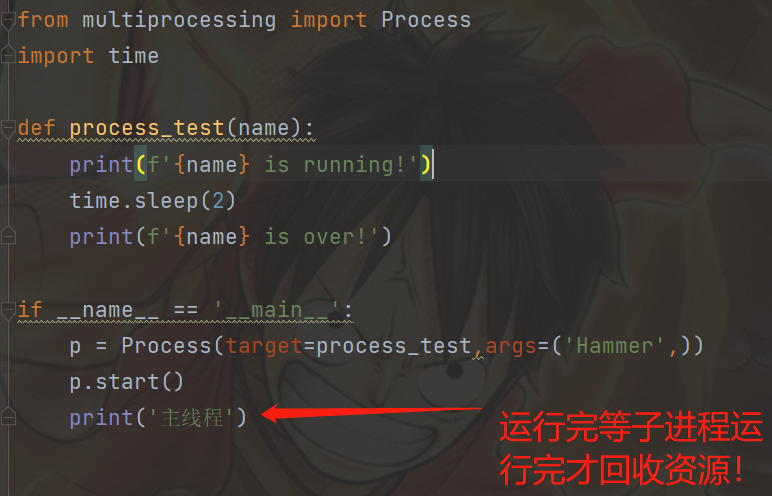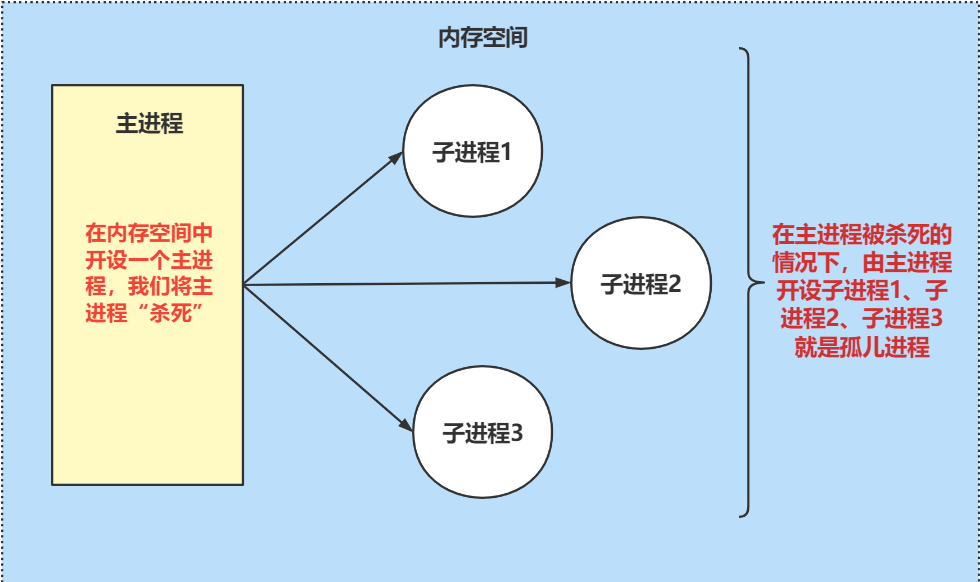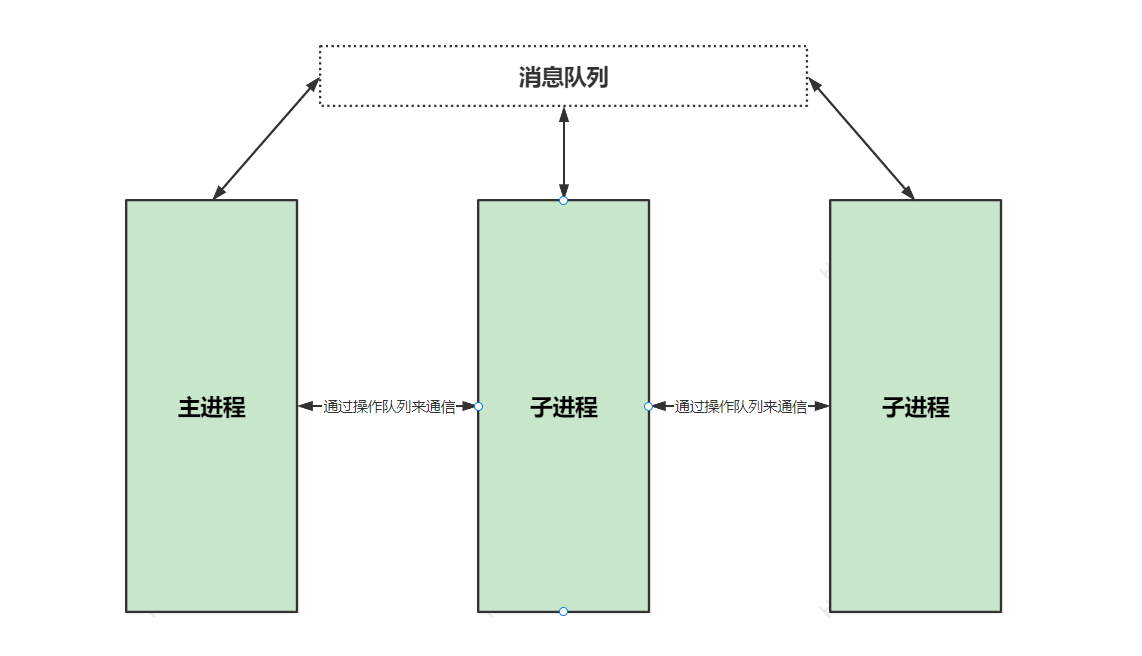# 并发编程（二）

## 僵尸进程``````from multiprocessing import Process
import time

def process_test(name):
print(f'{name} is running!')
time.sleep(2)
print(f'{name} is over!')

if __name__ == '__main__':
p = Process(target=process_test,args=('Hammer',))
p.start()
print('主线程')

# 主进程执行了print输出语句已经没有代码可以执行了，但是子进程是在该进程内开设的，需要等待回收子进程的资源
``````## 孤儿进程## 守护进程

``````from multiprocessing import Process
import time

def test(name):
print('总管:%s is running' % name)
time.sleep(3)
print('总管:%s is over' % name)

if __name__ == '__main__':
p = Process(target=test, args=('Hammer',))
p.daemon = True  # 设置为守护进程(一定要放在start语句上方)
p.start()
print("皇帝寿终正寝")
# 只有主进程的print语句，而子进程的输出语句不会执行
# 当主进程结束了，子进程也随即结束，就相当于皇帝死了，太监们就别玩了，而是去陪葬
``````

## 互斥锁

``````'''data.txt'''
{"ticket_num":1}

import json
from multiprocessing import Process, Lock
import time
import random

# 查票
def search(name):
with open(r'data.txt', 'r', encoding='utf8') as f:
ticket_num = data_dict.get('ticket_num')
print('%s查询余票:%s' % (name, ticket_num))

# 买票
# 先查票
with open(r'data.txt', 'r', encoding='utf8') as f:
ticket_num = data_dict.get('ticket_num')
# 模拟一个延迟
time.sleep(random.random())
# 判断是否有票
if ticket_num > 0:
# 将余票减一
data_dict['ticket_num'] -= 1
# 重新写入数据库
with open(r'data.txt', 'w', encoding='utf8') as f:
json.dump(data_dict, f)
print('%s: 购买成功' % name)
else:
print('不好意思 没有票了!!!')

def run(name,mutex):
search(name)
mutex.acquire()  # 抢锁
mutex.release()  # 释放锁
# 只需将买票上锁

# 开设多个进程
if __name__ == '__main__':
mutex = Lock()  # 多进程如果不上锁就会出现数据错乱
for i in range(1, 11):
p = Process(target=run, args=('用户%s' % i,mutex))
p.start()

# 抢锁的概念就相当于十个人去抢一个茅坑，一个人拉上了，后面的人只能等着了，拉完了（释放锁），别人才能继续抢锁;

# 在以后的编程生涯中 几乎不会解除到自己操作锁的情况
``````

## 乐观锁与悲观锁

### 乐观锁#

• 总是假设最好的情况，每次去拿数据的时候都认为别人不会修改，所以不会上锁，但是在更新的时候会判断一下在此期间别人有没有去更新这个数据，可以使用版本号机制和CAS算法实现。乐观锁适用于多读的应用类型，这样可以提高吞吐量，像数据库提供的类似于write_condition机制，其实都是提供的乐观锁；
• 乐观锁：假设不会发生并发冲突，只在提交操作时检查是否违反数据完整性；
• 乐观锁：多数用于数据争用不大、冲突较少的环境中，这种环境中，偶尔回滚事务的成本会低于读取数据时锁定数据的成本，因此可以获得比其他并发控制方法更高的吞吐量。
相对于悲观锁，在对数据库进行处理的时候，乐观锁并不会使用数据库提供的锁机制。一般的实现乐观锁的方式就是记录数据版本；

### 悲观锁#

• 总是假设最坏的情况，每次去拿数据的时候都认为别人会修改，所以每次在拿数据的时候都会上锁，这样别人想拿这个数据就会阻塞直到它拿到锁（共享资源每次只给一个线程使用，其它线程阻塞，用完后再把资源转让给其它线程，和Python中GIL锁一样）。传统的关系型数据库里边就用到了很多这种锁机制，比如行锁，表锁等，读锁，写锁等，都是在做操作之前先上锁；

• 悲观锁：假定会发生并发冲突，屏蔽一切可能违反数据完整性的操作；

• 悲观锁：主要用于数据争用激烈的环境，以及发生并发冲突时使用锁保护数据的成本要低于回滚事务的成本的环境中；

## 消息队列

``````from multiprocessing import Queue

# 括号内可以填写最大的等待数，队列最多装5个数
q = Queue(5)

# put方法，存放数据
q.put(111)
q.put(222)
print(q.full())  # 判断队列是否放满
q.put(333)
q.put(444)
q.put(555)
print(q.full())  # 队列放满了

# 超出范围原地等待，直到有空缺位置
# q.put(666)

# 提取数据
print(q.get())
print(q.get())
print(q.get())
print(q.get())
print(q.get())

# 只有五个数，取六个的时候，原地等待，直到有数据过来
# print(q.get())

# 判断数据是否为空
print(q.empty())
# 获取数据，没有数据立刻报错
print(q.get_nowait())  # queue.Empty
``````

• `full``get_nowait`不能用于多进程情况下的精确使用如果另外进程从当前进程拿数据，或者存入数据，会造成数据的偏差，从而`full方法``get_nowait方法`判断就会出错；

• 队列的使用就可以打破进程间默认无法通信的情况，这样就相当于开拓了一块公共空间（消息队列），每个进程都可以从中拿或存数据；

• [消息队列推荐博文](消息队列（mq）是什么？ - 知乎 (zhihu.com))

## IPC机制``````# 主进程和子进程通过消息队列来实现数据互通

# IPC机制来解决进程间无法通信的问题
from multiprocessing import Queue,Process

def consumer(q):
print(q.get())  # 子进程获取主进程存放的数据
q.put("子进程存放的数据")   # 模拟开设的子进程中存放的数据

if __name__ == '__main__':
q = Queue()  # 生成队列
q.put("主进程存放的数据") # 模拟数据
# 开设子进程
p = Process(target=consumer,args=(q,))
p.start()
p.join()  # 用join方法来确认子进程执行完，主进程再去取数据
print(q.get())  # 主进程获取
print("主进程")
``````
``````# 子进程和子进程通过消息队列来实现数据互通
from multiprocessing import Queue,Process

def process1(q):
q.put("子进程1存放的数据！")

def process2(q):
print(f'子进程2取子进程1存放的数据:>>>{q.get()}')

if __name__ == '__main__':
q = Queue()
p1 = Process(target=process1,args=(q,))
p2 = Process(target=process2,args=(q,))
p1.start()
p2.start()
``````

## 生产者消费者模型

👉[23种设计模型参考博文]((3条消息) Python之23种设计模式_Burgess_zheng的博客-CSDN博客_python 设计模式)

• 生产者：负责产生数据
• 消费者：负责处理数据

``````# 使用到模块JoinableQueue，能够监测谁给队列中放数据和取数据
from multiprocessing import Queue, Process, JoinableQueue
import time
import random

def producer(name, food, q):
for i in range(10):
print('%s 生产了 %s' % (name, food))
q.put(food)
time.sleep(random.random())

def consumer(name, q):
while True:
data = q.get()
print('%s 吃了 %s' % (name, data))

if __name__ == '__main__':
# q = Queue()
q = JoinableQueue()   # 队列模块换一下
p1 = Process(target=producer, args=('厨子Hammer', '玛莎拉', q))
p2 = Process(target=producer, args=('印度阿三', '飞饼', q))
p3 = Process(target=producer, args=('泰国阿人', '榴莲', q))
c1 = Process(target=consumer, args=('班长阿飞', q))

p1.start()
p2.start()
p3.start()
c1.daemon = True   # 守护进程
c1.start()

p1.join()
p2.join()
p3.join()

q.join()  # 等待队列中所有的数据被取干净

print('主进程')

# 使用模块JoinableQueue并且添加守护进程和join方法是为了让消费者结束等待的情况
``````